## Eisenstein Integer

The numbers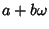, whereis one of the Roots of, the others being 1 and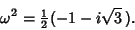Eisenstein integers are members of the Quadratic Field, and the Complex Numbers. Every Eisenstein integer has a unique factorization. Specifically, any Nonzero Eisenstein integer is uniquely the product of Powers of,, and the positive'' Eisenstein Primes (Conway and Guy 1996). Every Eisenstein integer is within a distanceof some multiple of a given Eisenstein integer.

Dörrie (1965) uses the alternative notation(1)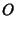(2)

forand, and calls numbers of the formG-Number.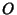and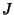satisfy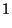(3)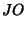(4)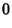(5)(6)(7)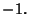(8)

The sum, difference, and products of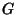numbers are alsonumbers. The norm of anumber is(9)

The analog of Fermat's Theorem for Eisenstein integers is that a Prime Number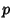can be written in the formIff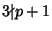. These are precisely the Primes of the form(Conway and Guy 1996).

References

Conway, J. H. and Guy, R. K. The Book of Numbers. New York: Springer-Verlag, pp. 220-223, 1996.

Cox, D. A. §4A in Primes of the Form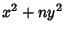: Fermat, Class Field Theory and Complex Multiplication. New York: Wiley, 1989.

Dörrie, H. The Fermat-Gauss Impossibility Theorem.'' §21 in 100 Great Problems of Elementary Mathematics: Their History and Solutions. New York: Dover, pp. 96-104, 1965.

Guy, R. K. Gaussian Primes. Eisenstein-Jacobi Primes.'' §A16 in Unsolved Problems in Number Theory, 2nd ed. New York: Springer-Verlag, pp. 33-36, 1994.

Riesel, H. Appendix 4 in Prime Numbers and Computer Methods for Factorization, 2nd ed. Boston, MA: Birkhäuser, 1994.

Wagon, S. Eisenstein Primes.'' Mathematica in Action. New York: W. H. Freeman, pp. 278-279, 1991.